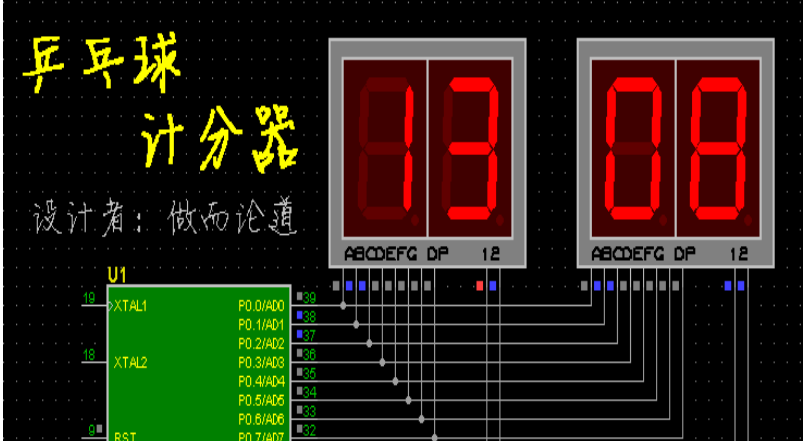# 51 单片机编程：双路计数器详解

+关注#include《reg51.h》

unsigned char n1， n2;

//-----------------------------------------------

void delay（unsigned int i） //1ms延时程序

{

unsigned char j;

while（i--） for（j = 0; j 《 115; j++）;

}

//-----------------------------------------------

void disp（void）

{

unsigned char code SEG［］ = {

0xc0， 0xf9， 0xa4， 0xb0， 0x99， //0-4

0x92， 0x82， 0xf8， 0x80， 0x90};//5-9

P0 = SEG［n1 / 10］; P1 = 1; delay（2）; P1 = 0;

P0 = SEG［n1 % 10］; P1 = 2; delay（2）; P1 = 0;

P0 = SEG［n2 / 10］; P1 = 4; delay（2）; P1 = 0;

P0 = SEG［n2 % 10］; P1 = 8; delay（2）; P1 = 0;

}

//-----------------------------------------------

void main（void）

{

unsigned char k， t;

n1 = n2 = 0;

while（1） {

disp（）;

if （~（P2 | 0xc0）） {

disp（）;

if （~（P2 | 0xc0）） {

k = ~（P2 | 0xc0）;

while （~（P2 | 0xc0）） disp（）;

if （k == 1） {n1++; if （n1 == 100） n1 = 0;}

else if （k == 2） {n1--; if （n1 == -1） n1 = 99;}

else if （k == 4） {n2++; if （n2 == 100） n2 = 0;}

else if （k == 8） {n2--; if （n1 == -1） n2 = 99;}

else if （k == 16） {n1 = n2 = 0;}

else if （k == 32） {t = n1; n1 = n2; n2 = t;}

} } }

}

//-----------------------------------------------

ORG 0000H

JMP MAIN

ORG 0030H

;-------------------------

MAIN：

CALL DISP

MOV A， P2

CJNE A， #0FFH， KEY

CLR 00H

JMP MAIN

;-------------------------

KEY：

JMP MAIN

;----------------------

SETB 00H

JB P2.0， A_SUB1

MOV A， R0

DA A

MOV R0， A

JMP MAIN

;----------------------

A_SUB1：

MOV A， R0

DA A

MOV R0， A

JMP MAIN

;----------------------

JB P2.2， B_SUB1

MOV A， R1

DA A

MOV R1， A

JMP MAIN

;----------------------

B_SUB1：

JB P2.3， CLR_0

MOV A， R1

DA A

MOV R1， A

JMP MAIN

;----------------------

CLR_0：

JB P2.4， EXCH

MOV R0， #00H

MOV R1， #00H

JMP MAIN

;----------------------

EXCH：

JB P2.5， END_K

MOV A， R0

XCH A， R1

MOV R0， A

JMP MAIN

END_K：

CLR 00H

JMP MAIN

;-------------------------

DISP：

MOV DPTR， #BG

MOV A， R0

SWAP A

ANL A， #0FH

MOVC A， @A + DPTR

MOV P0， A

SETB P1.0

CALL DELAY

CLR P1.0

;----------------------

MOV A， R0

ANL A， #0FH

MOVC A， @A + DPTR

MOV P0， A

SETB P1.1

CALL DELAY

CLR P1.1

;----------------------

MOV A， R1

SWAP A

ANL A， #0FH

MOVC A， @A + DPTR

MOV P0， A

SETB P1.2

CALL DELAY

CLR P1.2

;----------------------

MOV A， R1

ANL A， #0FH

MOVC A， @A + DPTR

MOV P0， A

SETB P1.3

CALL DELAY

CLR P1.3

RET

;-------------------------

DELAY：

DJNZ R7， \$

DJNZ R7， \$

DJNZ R7， \$

DJNZ R7， \$

RET

;-------------------------

BG：

DB 0C0H，0F9H，0A4H，0B0H， 99H

DB 92H， 82H，0F8H， 80H， 90H

;-------------------------

END